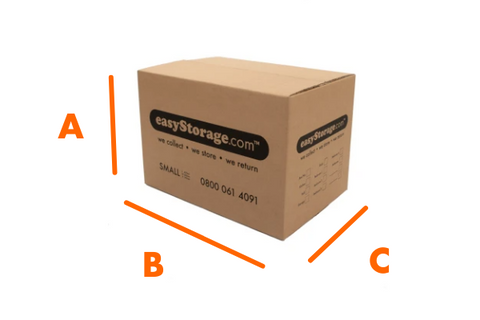# How to calculate the volume of a Moving Box

Don't feel stress out just by the thought of how you can fit all your items in a moving box. There is a wide range of sizes out there and I guarantee you, you can find the perfect match for your needs.

There are many occasions when we do not just need to know the measurements of the moving box, but also the volume and/or capacity it can hold.

In this post we will show you how to calculate the volume of a box in litres, as this is the quickest and the simplest formula for calculating the volume. You only need to know the measurements of the box and, very IMPORTANT, that they are all in the same unit of measurement (cm, mm, m...).

# Calculate the Volume

## 1. First Steps

We draw a simulation of one of our boxes and mark it out with the measurements.A. Height

B. Length

C. Width

## 2. Formula to calculate the volume:

The way to calculate it, as we said, is very simple with this simple formula.

HEIGHT x LENGTH x WIDTH = X cm3

For example, how can we find the volume of our box if it is 60x40x20 cm?

60x40x20x20=48000 cm3.

## 3. Other measurements:

cm3 to m3, volume to litres, volume to kg.

### M3

Bearing in mind that 1 cubic metre = 1000000 cubic centimetres we can easily convert this measurement to m3.

48000/1000000= 0.048 m3

### LITRES

Sometimes we need to know the measures of volume to litres, taking into account that 1cm3=0.001 L or 1L=1000cm3

48000/1000=48 litres

### KG

This value will be exactly the same as the litres since 1L=1Kg.

We hope this post has helped you to calculate the volume to decide your ideal packaging supplies. If you have any questions, please contact our team, and we will be delighted to help you.